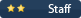# ydoaPs

Moderators

10562

• #### Days Won

1

ydoaPs had the most liked content!

## Community Reputation• Rank
The Oncoming Storm
• Birthday 04/21/1988

## Profile Information

• Location
Local Group
• College Major/Degree
BA in Philosophy, pursuing MA in History and Philosophy of Physics
• Favorite Area of Science
Physics
• Biography
...
• Occupation
Former Nuclear Mechanic; Current Philosopher

• Skype
ydoaPs

## Recent Profile Visitors

88995 profile views

• ### nevim

1.## Irreducible complexity (split from Could intelligent design be legitimate?)

The Muller Two Step was a prediction of evolution before Behe was even born, let alone before he decided it was somehow a problem for evolution.
2. And that's not even counting all of the other forms of sameness like adjoint situations. There's so many different forms of "eh, that's sort of the same as this other thing in certain ways".
3. What LaTeX packages can we use in blogs? If the one we need (say, for commutative diagrams) isn't installed, is there a way to get it installed?
4. If I'm understanding you correctly, I think you're using different terminology. It might be a geographic thing. As far as I learned, an Equivalence Relation has the properties you detailed in your post, and, by the process I discussed, partitions a set into Equivalence Classes resulting in the quotient set (or monoid or group or category etc). I'm not sure what there is to gain by using "equivalence" only in terms of equivalence classes rather than for either equivalence relations and equivalence classes. If we had to pick, I'd probably make the relation "Equivalence", but that's probably
5.## What made you stop believing in God?

Jesus also got mad when a fig tree didn't produce figs when it was not fig season.
6. That's a great post. Yeah, equivalence relations are much looser than identity. Any bivalent relation will give you an equivalence relation. You can actually even define an equivalence relation out of any function. Take a function f, and you can define the equivalence relation ~ such that a~b iff f(a)~f(b). This is part of a process of decomposing a function. If the domain (what the function is taking as an argument) is A, and the the codomain (what the function points to) is B, then you can decompose a function via ~ in the following way. Make a function f* that takes A and map
7. How many coin flips does it take to make a free choice?
8.## Starting Python question on where to begin...

You'll need to define a function; do you know how? You'll need to take an input from the user; Do you know how? You'll need to make sure the data type of the input is a certain type; do you know what type that is and how to check that the input is that type? Then you'll need to perform the calculation and print the result; Do you know how? Give it a shot, and if you can't figure out how to do any of the above steps, let us know. We won't do your homework for you, but we'll help you figure it out.
9.## Navy admits UFOs are inexplicable!

That's kind of the definition of UFO.
10.## How does one represent the fourth dimension on a graph?

You could map the wavelengths of visible light to [0,1], map [0,1] to some continuous subset of your time variable, then do a graph for different colors (points in time) for any "granularity" of colors (moments) that you'd like.
11.## Can Something Exist for Zero Seconds?

Throwing a ball up has it start with a velocity v coming out of your hand. There is no force causing it to accelerate upward, but there is a force causing it to accelerate downward: gravity. So, from v, the velocity slows with the acceleration due to gravity until it stops. But, then, it starts going down, still accelerating due to gravity. This trajectory is symmetric about the point at the top where the velocity is zero. So, for any other point, there is a partner where the ball has the same speed. But for each speed, the ball has that velocity for 0 seconds. There must be a time o
12.## Can Something Exist for Zero Seconds?

For any velocity the ball has along its trajectory, it has it for precisely 0 seconds, and for all but v_y = 0, it has said velocity twice for 0 seconds.
13.## Can Something Exist for Zero Seconds?

Precisely 0. There must be a time where v_y=0, but the amount of time v_y=0 is precisely 0. This is honestly very unintuitive for a lot of people (Iirc, this might explicitly be a motivating example in the intro to Frames and Locales). Tbh, this is a big part of why I'm not a fan of pointy space. Localic space ftw.
14.## Can Something Exist for Zero Seconds?

! Moderator Note Topic moved to General Philosophy
15. ! Moderator Note Topic moved to General Philosophy
×